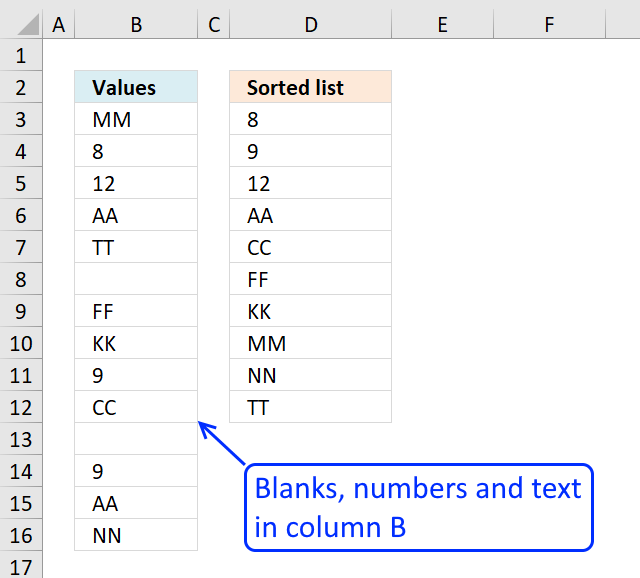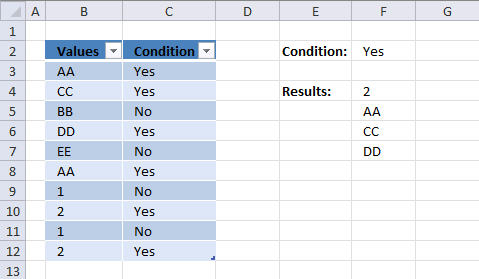Author: Oscar Cronquist Article last updated on December 10, 2020The image above demonstrates a formula in cell D3 that extracts unique distinct numbers and text values sorted from A to Z and also ignoring blank cells.

Array formula in cell D3:

=IFERROR(SMALL(IF((COUNTIF(\$D\$2:D2, \$B\$3:\$B\$16)=0)*ISNUMBER(\$B\$3:\$B\$16), \$B\$3:\$B\$16, "A"), 1), INDEX(\$B\$3:\$B\$16, MATCH(SMALL(IF(ISTEXT(\$B\$3:\$B\$16)*(COUNTIF(D2:\$D\$2, \$B\$3:\$B\$16)=0), COUNTIF(\$B\$3:\$B\$16, "<"&\$B\$3:\$B\$16), ""), 1), IF(ISTEXT(\$B\$3:\$B\$16), COUNTIF(\$B\$3:\$B\$16, "<"&\$B\$3:\$B\$16), ""), 0)))

Update 10th of December 2020: Excel 365 subscribers can now use this much shorter regular formula.

=SORT(UNIQUE(FILTER(B3:B16,B3:B16<>"")))

Here is how it works:Extract unique distinct values sorted from A to Z ignoring blanks

How to create an array formula

1. Copy above array formula
2. Select cell D3
3. Click in formula bar4. Paste formula (Ctrl + v)
5. Press and hold Ctrl + Shift
6. Press Enter

How to copy array formula

Copy cell B2 and paste it down as far as needed.

### Explaining formula in cell D3

There are two formulas in the IFERROR function, when the first formula is running out of numbers to return the second formula starts extracting text values.

IFERROR( formula1, formula2)

#### Step 1 - Prevent extracting duplicate numbers

The COUNTIF function counts cells in cell range based on a condition or criteria. If the value is equal to 0 then it has not been displayed yet. The first cell reference grows when the cell is copied to cells below, this makes the formula aware of previously displayed value above the current cell.

COUNTIF(\$D\$2:D2,\$B\$3:\$B\$16)=0)

becomes

COUNTIF("Sorted list",{"MM"; 8; 12; "AA"; "TT"; 0; "FF"; "KK"; 9; "CC"; 0; 9; "AA"; "NN"})=0

becomes

{0;0;0;0;0;0;0;0;0;0;0;0;0;0}=0

and returns

{TRUE; TRUE; TRUE; TRUE; TRUE; TRUE; TRUE; TRUE; TRUE; TRUE; TRUE; TRUE; TRUE; TRUE}

#### Step 2 - Identify numbers in column

The ISNUMBER function returns TRUE if value is a number.

ISNUMBER(\$B\$3:\$B\$16)

becomes

ISNUMBER({"MM"; 8; 12; "AA"; "TT"; 0; "FF"; "KK"; 9; "CC"; 0; 9; "AA"; "NN"})

and returns

{FALSE; TRUE; TRUE; FALSE; FALSE; FALSE; FALSE; FALSE; TRUE; FALSE; FALSE; TRUE; FALSE; FALSE}

#### Step 3 - Multiply arrays

Both arrays must return TRUE for the logical expression to return TRUE. In ordeer to achieve AND logic I multiply the arrays. TRUE * TRUE = TRUE, TRUE * FALSE = FALSE and FALSE * FALSE = FALSE.

(COUNTIF(\$D\$2:D2, \$B\$3:\$B\$16)=0)*ISNUMBER(\$B\$3:\$B\$16)

becomes

{TRUE; TRUE; TRUE; TRUE; TRUE; TRUE; TRUE; TRUE; TRUE; TRUE; TRUE; TRUE; TRUE; TRUE}*{FALSE; TRUE; TRUE; FALSE; FALSE; FALSE; FALSE; FALSE; TRUE; FALSE; FALSE; TRUE; FALSE; FALSE}

and returns

{0;1;1;0;0;0;0;0;1;0;0;1;0;0}. 0 (zeros is the equivalent to FALSE and any other number is equal to boolean TRUE.

#### Step 4 - Replace TRUE with numbers in column

The IF function returns one value (argument2) if TRUE and another (argument3) if FALSE. The IF function returns "A" if logical expression is FALSE, this makes the SMALL function ignore the text value in the next step.

IF((COUNTIF(\$D\$2:D2, \$B\$3:\$B\$16)=0)*ISNUMBER(\$B\$3:\$B\$16), \$B\$3:\$B\$16, "A")

becomes

IF({0;1;1;0;0;0;0;0;1;0;0;1;0;0}, \$B\$3:\$B\$16, "A")

becomes

IF({0;1;1;0;0;0;0;0;1;0;0;1;0;0}, {"MM"; 8; 12; "AA"; "TT"; 0; "FF"; "KK"; 9; "CC"; 0; 9; "AA"; "NN"}, "A")

and returns {"A";8;12;"A";"A";"A";"A";"A";9;"A";"A";9;"A";"A"}.

#### Step 5 - Extract smallest number

To be able to return a new value in a cell each I use the SMALL function to filter numbers from smallest to largest.

SMALL(IF((COUNTIF(\$D\$2:D2, \$B\$3:\$B\$16)=0)*ISNUMBER(\$B\$3:\$B\$16), \$B\$3:\$B\$16, "A"), 1)

becomes

SMALL({"A";8;12;"A";"A";"A";"A";"A";9;"A";"A";9;"A";"A"}, 1)

and returns 8 in cell D3.

### Explaining formula in cell D6

#### Step 1 - Prevent duplicates and only extract text values

This step and forward explains how to extract text values. We begin with cell D6 which is the first cell that extracts text values in our example. The ISTEXT function returns TRUE if value is a text value. The IF function returns a number representing the rank order if the list were sorted from A to Z.

IF(ISTEXT(\$B\$3:\$B\$16)*(COUNTIF(D\$2:\$D5, \$B\$3:\$B\$16)=0), COUNTIF(\$B\$3:\$B\$16, "<"&\$B\$3:\$B\$16), "")

becomes

IF({1;0;0;1;1;0;1;1;0;1;0;0;1;1}, COUNTIF(\$B\$3:\$B\$16, "<"&\$B\$3:\$B\$16), "")

becomes

IF({1; 0; 0; 1; 1; 0; 1; 1; 0; 1; 0; 0; 1; 1}, {5; 0; 3; 0; 7; 0; 3; 4; 1; 2; 0; 1; 0; 6}, "")

and returns

{5; ""; ""; 0; 7; ""; 3; 4; ""; 2; ""; ""; 0; 6}.

#### Step 2 - Extract k-th smallest number

SMALL(IF(ISTEXT(\$B\$3:\$B\$16)*(COUNTIF(D\$2:\$D5, \$B\$3:\$B\$16)=0), COUNTIF(\$B\$3:\$B\$16, "<"&\$B\$3:\$B\$16), ""), 1)

becomes

SMALL({5; ""; ""; 0; 7; ""; 3; 4; ""; 2; ""; ""; 0; 6}, 1)

and returns 0 (zero).

#### Step 3 - Find number in array

The MATCH function finds the relative position of a value in an array or cell range.

MATCH(SMALL(IF(ISTEXT(\$B\$3:\$B\$16)*(COUNTIF(D\$2:\$D5, \$B\$3:\$B\$16)=0), COUNTIF(\$B\$3:\$B\$16, "<"&\$B\$3:\$B\$16), ""), 1), IF(ISTEXT(\$B\$3:\$B\$16), COUNTIF(\$B\$3:\$B\$16, "<"&\$B\$3:\$B\$16), ""), 0)

becomes

MATCH(0, IF(ISTEXT(\$B\$3:\$B\$16), COUNTIF(\$B\$3:\$B\$16, "<"&\$B\$3:\$B\$16), ""), 0)

becomes

MATCH(0, {5; ""; ""; 0; 7; ""; 3; 4; ""; 2; ""; ""; 0; 6}, 0)

and returns 4.

#### Step 4 - Extract value based on position

The INDEX function returns a value based on a cell reference and column/row numbers.

INDEX(\$B\$3:\$B\$16, MATCH(SMALL(IF(ISTEXT(\$B\$3:\$B\$16)*(COUNTIF(D\$2:\$D5, \$B\$3:\$B\$16)=0), COUNTIF(\$B\$3:\$B\$16, "<"&\$B\$3:\$B\$16), ""), 1), IF(ISTEXT(\$B\$3:\$B\$16), COUNTIF(\$B\$3:\$B\$16, "<"&\$B\$3:\$B\$16), ""), 0))

becomes

INDEX(\$B\$3:\$B\$16, 4)

and returns "AA" in cell D6.

### Download Excel fileUnique-and-Sort-numbers-and-text-cells-using-excel-array-formula.xlsx

### Create a unique distinct sorted list containing both numbers text removing blanks with a conditionArray formula in cell F4:

=IFERROR(INDEX(Table1[Values], MATCH(SMALL(IF(Table1[Condition]=\$F\$2, IF(ISBLANK(Table1[Values]), "", IF(COUNTIF(\$F\$3:F3, Table1[Values])=0, IF(ISNUMBER(Table1[Values]), COUNTIF(Table1[Values], "<"&Table1[Values]), COUNTIF(Table1[Values], "<"&Table1[Values])+SUM(1*ISNUMBER(Table1[Values]))+1), "")), ""), 1), IF(Table1[Condition]=\$F\$2, IF(ISBLANK(Table1[Values]), "", IF(COUNTIF(\$F\$3:F3, Table1[Values])=0, IF(ISNUMBER(Table1[Values]), COUNTIF(Table1[Values], "<"&Table1[Values]), COUNTIF(Table1[Values], "<"&Table1[Values])+SUM(1*ISNUMBER(Table1[Values]))+1), "")), ""), 0)), "")

How to create an array formula

How to copy array formula in cell F4

1. Select cell F4
2. Copy (Ctrl + c)
3. Select cell range F5:F10
4. Paste (Ctrl + v)

### Download Excel fileunique-list-sorted-alphabetically-with-a-condition1.xlsx